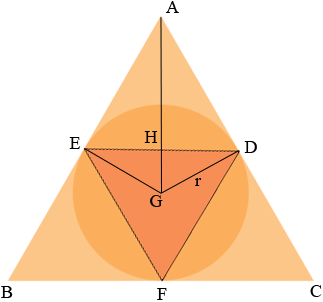SEARCH HOMEMath Central Quandaries & QueriesQuestion from Anita, a teacher: An equilateral triangle with side of length 1 cm is inscribed in a circle. A second equilateral triangle is circumscribed about the circle with all sides tangent to the circle. Find the length of a side of the second triangle.Hi Anita,Since you can rotate the circle inside the larger triangle without changing any of the dimensions I chose to have the vertices of the smaller triangle at the points of tangency of the sides of larger triangle to the circle.

The measure of angle EFD is 60o and this angle is subtended by the chord ED. But ED subtends the angle EGD at the centre of the circle so the measure of the angle EGD is 120o and the measure of the angle HGD is therefore 60o. Angle GDA is a right angle so the measure of angle DAG is 30o.

Triangle AHD is a right triangle and hence it is a 30-60-90 triangle. Thus 2|HD| = |AD| and thus

s = 2|AD| = 4|HD| = 4(1/2) = 2.

HarleyMath Central is supported by the University of Regina and The Pacific Institute for the Mathematical Sciences.